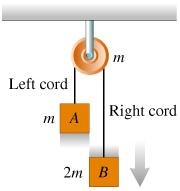# Problem: The figure below shows two blocks suspended by a cord over a pulley. The mass of block B is twice the mass of block A, while the mass of the pulley is equal to the mass of block A. The blocks are let free to move and the cord moves on the pulley without slipping or stretching. There is no friction in the pulley axle, and the cord's weight can be ignored. Which of the following statements correctly describes the system shown in the figure?A. The acceleration of the blocks is zeroB. The net torque on the pulley is zeroC. The angular acceleration of the pulley is non-zero

###### FREE Expert Solution

Tension in the rope is uniform. That is, the tension on the left side is equal to the tension on the left side.

90% (94 ratings)###### Problem Details

The figure below shows two blocks suspended by a cord over a pulley. The mass of block B is twice the mass of block A, while the mass of the pulley is equal to the mass of block A. The blocks are let free to move and the cord moves on the pulley without slipping or stretching. There is no friction in the pulley axle, and the cord's weight can be ignored. Which of the following statements correctly describes the system shown in the figure?A. The acceleration of the blocks is zero

B. The net torque on the pulley is zero

C. The angular acceleration of the pulley is non-zero

Frequently Asked Questions

What scientific concept do you need to know in order to solve this problem?

Our tutors have indicated that to solve this problem you will need to apply the Torque & Acceleration (Rotational Dynamics) concept. You can view video lessons to learn Torque & Acceleration (Rotational Dynamics). Or if you need more Torque & Acceleration (Rotational Dynamics) practice, you can also practice Torque & Acceleration (Rotational Dynamics) practice problems.

What professor is this problem relevant for?

Based on our data, we think this problem is relevant for Professor Evrard's class at UMICH.